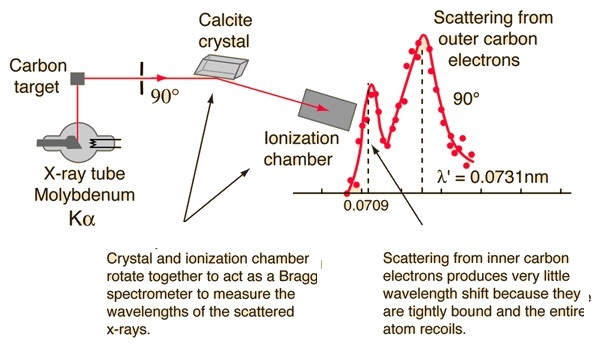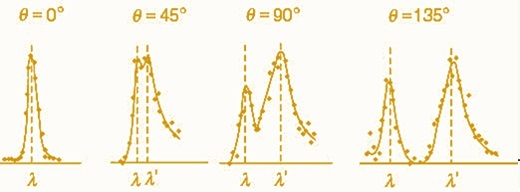# Compton Shift

## Introduction

Compton shift explains the Compton scattering of electromagnetic radiations. Visible light is electromagnetic radiation and is the only part of the electromagnetic spectrum that can be visible to the human eye. Photon is the basic unit of light. It is a quantum of energy or energy packet in electromagnetic radiation. Albert Einstein explained the fact of discrete energy packets during the transmission of light. And it was first named a photon by Gilbert N. Lewis. They are always in motion and move with the speed of light in a vacuum. All electromagnetic energy is made up of photons.

## What is the Compton effect?

When energetic electromagnetic radiation such as X - rays undergoes elastic scattering by a charged particle such as an electron, the wavelength of the X-ray is increased. This is called the Compton effect . It is also called Compton scattering. It is one of the important phenomena in quantum mechanics which is responsible for the study of wave and particle properties of radiation and also matter.

This increase in wavelength of the X - rays undergoing elastic scattering was explained by an American physicist named Arthur Holly Compton in 1922. He said that the X - rays are composed of quanta or discrete pulses of electromagnetic radiation. Later the American chemist Gilbert Lewis named the quanta of radiation photons.

## What is Compton’s shift?

The photons give their energy to electrons which are at rest while they collide with each other. Due to this the wavelength of the scattered electron is increased. This increase in the wavelength of the photon is called the Compton shift. As there is a relationship between energy and wavelength of the photon, the longer wavelength of the scattered photon depends on the angle through which the incident X-ray gets diverted. The wavelength of the incident photon does not influence the Compton shift. The difference between the wavelength of the incident photon and the scattered photon is given by,

$$\mathrm{\lambda'-\lambda=(\frac{h}{mc})(1-cos\theta)}$$

Here, h - denotes Planck’s constant.

m - denotes the rest mass of the electron

c - denotes the speed of light

θ - denotes the angle through which the photon is scattered.

## Example

Let us consider the Compton effect of molybdenum $\mathrm{K_\alpha\:X-ray}$. Let the wavelength of the X-ray of that targeted graphite be 0.0709 nm. There occur two peaks which are due to the scattering of photons from the inner and outer electrons of carbon atoms. The first peak is low because the photons interact with the tightly bounded inner electrons of the carbon atom. It is hard to free the electron. So, the Compton shift is negligible. The second peak is high as it is due to the interaction of photons with the loosely bounded outer electrons of carbon. As they are loosely bound the photon gives some of its energy to the electron and frees it.If the scattering angle is increased, then Compton shift also increases because of the drop in energy of the photon that is scattered. The Compton shift for different angles of scattering is given below.## Derivation for Compton Shift Equation

Consider a photon colliding with an electron elastically.

$$\mathrm{Momentum\:p=\frac{h u}{c}}$$

According to the conservation of momentum

For horizontal component

$$\mathrm{p=p'cos\phi+p_e cos\phi}$$

$$\mathrm{p_e cos\phi=p-p'cos\phi}$$

For vertical component

$$\mathrm{0=p'sin\theta-p_e sin\phi}$$

$$\mathrm{p_e sin\theta=p'sin\theta}$$

Combining these two-equations

$$\mathrm{p^2_e cos^2 \phi+p^2_e sin^2 \phi=(p-p'cosθ)^2+p^{,2} sin^2 \theta}$$

As we know $\mathrm{sin^2 \phi+cos^2 \phi=1}$

$$\mathrm{p^2_e=p^2+p^{,2}-2pp'cos\theta}$$

According to conservation of energy

$$\mathrm{E+E_0=E'+E_e}$$

$$\mathrm{E=m^2 c^4+p^2 c^2\:and\:E=pc \:for\:photon}$$

$$\mathrm{pc+mc^2=p'c+(m^2 c^4+p^2 c^2 )^{1/2}}$$

$$\mathrm{p-p'+mc^2=m^2 c^2}$$

$$\mathrm{p^2+P'^2+m^2 c^2+2(-pp'+pmc+-p'mc)=m^2 c^2+p^2+p'^2-2pp'cos\theta}$$

$$\mathrm{pmc-p'mc=pp'(1-cos\theta)}$$

$$\mathrm{\frac{mc}{p'}-\frac{mc}{p}=1-cosθ}$$

$$\mathrm{\frac{mc\lambda}{h}-\frac{mc\lambda}{h}=1-cos\theta}$$

$$\mathrm{λ'-λ=\frac{h}{mc}(1-cos\theta)}$$

## Applications of Compton effect

### Compton scattering

• Compton effect is applicable in radiobiology. In radiation therapy the atoms present in the living beings are interacted with the high energy X - rays and gamma rays is applied.

• As the gamma rays can scatter out of the detector, Compton suppression is used to detect the scattered rays.

### Magnetic Compton Scattering

• When a crystal sample is hit by a high energy photon it gets magnetised. This technique is called magnetic Compton effect. There is a generation of two different Compton profiles. Magnetic Compton profile (MCP) is the difference between these two Compton profiles. It is an incoherent process.

• MCP is responsible for the bulk properties of the sample. The area under the MCP directly depends on the spin moment of the system.

• It is applied for measuring the spin specific magnetic hysteresis loop (SSMH).

### Inverse Compton Scattering

• Inverse Compton scattering is applied in astrophysics. A black hole that is surrounded by an accretion disk is thought to give a thermal spectrum. The high energy electrons in the corona scatter the low energy photons into high energy photons. It is applied in nuclear physics experiments.

### Non - Linear Compton Scattering

• During the interactions between the charged particle such as electron and photon low energy photons are scattered by an intense magnetic field. This is called Inverse Compton scattering.

• As non - linear Compton scattering is able to produce high energy photons, it is applied for the applications where there is a need for high energy photons.

## Conclusion

Light composed of a packet of energetic particles called photons. In this tutorial the facts about Compton effect and Compton shift were discussed in detail. The derivation for the Compton shift and the applications of the Compton effect were discussed above.

## FAQs

Q1. What are the properties of light?

Ans.

• Light energy can pass through the vacuum.

• Light moves at a velocity of about 3 x 108 m/s.

• Light undergoes reflection, refraction, diffraction, scattering, and polarisation.

• The wavelength of light changes when monochromatic light travels from one medium to another. But its frequency does not change.

Q2. What is a photon?

Ans. Photons are the basic unit of light. It is a quantum of energy or energy packet in electromagnetic radiation. They are always in motion and move with the speed of light in a vacuum. All electromagnetic energy is made up of photons. They are always neutral.

That is, they have no electric charge. They do not decay on their own. Energy of a photon is given by,

$$\mathrm{E=h u}$$

Q3. What are the differences between Compton effect and photoelectric effect?

Ans. Difference between Compton effect and Photoelectric effect

Compton EffectPhotoelectric Effect
It happens due to the high energy photons that are bound to free the electrons.It happens due to the weakly bound electrons that are emitted from the surface when they interact with electromagnetic radiation.
It was explained by Arthur ComptonIt was explained by Albert Einstein

Q4. In the Compton equation what should be substituted for mass?

Ans. In the Compton equation the value substituted for mass is the mass of an atom not the mass of the electron.

Q5. What does the Compton effect depend on?

Ans. Compton effect depends on electron density, density of the material and weakly on photon energy.

Updated on: 26-Apr-2023

449 Views